##### Quantum Physics For Dummies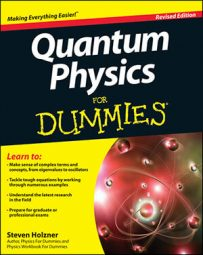You can create the actual eigenstates, | l, m >, of angular momentum states in quantum mechanics. When you have the eigenstates, you also have the eigenvalues, and when you have the eigenvalues, you can solve the Hamiltonian and get the allowed energy levels of an object with angular momentum.

Don't make the assumption that the eigenstates are | l, m >; rather, say they're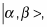where the eigenvalue of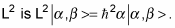So the eigenvalue of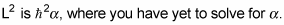Similarly, the eigenvalue of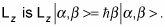To proceed further, you have to introduce raising and lowering operators. That way, you can solve for the ground state by, for example, applying the lowering operator to the ground state and setting the result equal to zero — and then solving for the ground state itself.

In this case, the raising operator is L+ and the lowering operator is L. These operators raise and lower the Lz quantum number. You can define the raising and lowering operators this way:

• Raising: L+ = Lx + iLy

• Lowering: L = LxiLy

These two equations mean that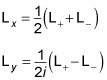You can also see that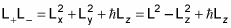That means the following are all equal to L2: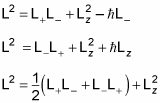You can also see that these equations are true: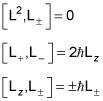Okay, now you can put all this to work. You're getting to the good stuff.

Take a look at the operation of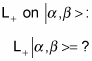To see what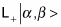is, start by applying the Lz operator on it like this: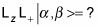From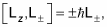you can see that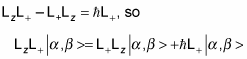And because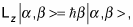you have the following: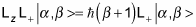This equation means that the eigenstate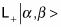is also an eigenstate of the Lz operator, with an eigenvalue of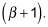Or in a more comprehensible way: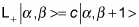where c is a constant.

So the L+ operator has the effect of raising the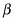quantum number by 1. Similarly, the lowering operator does this: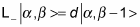Now take a look at what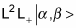equals: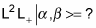Because L2 is a scalar, it commutes with everything. L2 L+ – L+ L2 = 0, so this is true: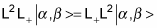And because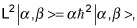you have the following equation: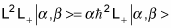Similarly, the lowering operator, L, gives you this: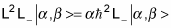So the results of these equations mean that the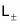operators don't change the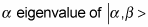at all.